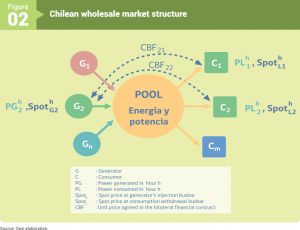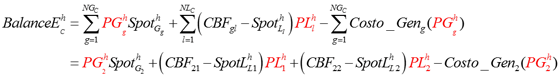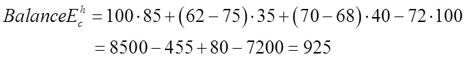Menú Principal

# Annex 2.1 Dispatch

The dispatch of units in the system is performed by the Coordinator, using optimization tools (economic dispatch, pre-dispatch, hydrothermal coordination) and determining system operation at minimum cost. This optimization also determines the hourly marginal cost (spot price) for each busbar of the system.

Figure 2 shows the general scheme of the wholesale electricity market in Chile.For a specific hour h, the pool establishes an economic operation of the system that gives rise to marginal costs at both injection and withdrawal busbars of the system (SpotG and SpotL). In its simplest form, the economic operation of the system is achieved dispatching generation units in ascending order of generation cost, until the required demand in a given hour can be covered. Thus, zero or low cost units are dispatched first. This type of generation, common among NCRE, is called base generation units.

Assuming that all consumption is previously contracted through CBFij-type financial contracts (Bilateral Financial Contracts), each company calculates its balance taking into account revenues for injections at the injection point, energy withdrawals for its customers and customer payments under the bilateral contract in force. Likewise, the generation company considers its variable generation costs in the balance.

The following equation summarizes the situation for generation company G2 that has bilateral contracts with consumers C1 and C2. At hour h, the generator injects into the system PG2 [MWh], while its contracted consumers withdraw PL1 [MWh] and PL2 [MWh], respectively.To illustrate the above, let us assume that G2 is a thermal coal-fired generator and at a specific hour (h) injects 100 MW into the system at a marginal cost at the injection busbar of 85 US\$/MW. The generator has agreed contracts for electricity sales with C1 and C2 for 62 US\$/MW and 70 US\$/MW, respectively. The Coordinator determines that the costs at purchase busbars L1 and L2 are 75 US\$/MW and 68 US\$/MW, while consumption are 35 and 40 MW, respectively. Finally, the variable cost of the generator is 72 US\$/MW. Therefore, the balance of G2 is equal to:The result of the balance is US\$ 925, and for the operating conditions of the example, G2 is surplus. However, there may be other situations when this is not the case, as occurs when its injections into the system are lower than its contractual commitments.